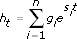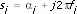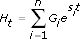# Building Modal Parametric Models (Advanced Signal Processing Toolkit)

LabVIEW 2014 Advanced Signal Processing Toolkit Help

Edition Date: June 2014

Part Number: 372656C-01

»View Product InfoDownload Help (Windows Only)

A modal parametric model describes the bulk dynamic characteristics of resonant systems such as buildings and bridges. The dynamic characteristics include natural frequencies, damping factors, resonance magnitudes, and resonance phases.

You can use the impulse response function to determine the response of a linear system to stimulus. The impulse response time series for a linear system can be represented parametrically with a linear combination of damped complex sinusoids as follows:where t is the time index n is the model order gi is one of the complex magnitudes si is one of the modal poles

si contains a unified frequency fi and a damping factor alphai as follows:The parameter gi contains a magnitude ri and a phase θias follows:So a discrete-time system can be defined by a series of resonance components, or modes: natural frequencies fi, damping factors alphai, resonance magnitudes ri, and resonance phases θi.

For a multivariate impulse response time series, you can build a modal parametric model as follows:where Ht is a (k × 1) vector with k variables that come from k sources. HtT = [h1t, h2t, ..., hkt]. Gi is a (k × 1) complex magnitude vector with k variables. GiT = [g1i, g2i, ..., gki]. Each variable in the vector Gi is one of the complex magnitudes. si is one of the modal poles.

## Specifying an Appropriate Model Order

The model order determines the number of modes that a modal parametric model contains. A model can contain real modes or pairs of complex conjugate modes. A real mode generates a resonance component at 0 Hz or at the Nyquist frequency. A pair of conjugate complex modes generates a resonance component with a positive frequency and discards the conjugate resonance component with the corresponding negative frequency. To search for m positive resonance components, you must specify the model order to be at least 2 × m. If a time series contains a large offset, you need to set the model order to at least 2 × m + 1 to allow for a real mode. Structural vibration time series typically do not have offsets, so you can use a model order of 2 × m.Note  Noise and distortion in the measured time series, as well as an insufficient model order, can result in the estimated model containing spurious components. To discern which components in the estimated model are valid, you can try specifying different model orders and form a histogram for the estimated resonance components. In the histogram, the true resonance components of the dynamic system typically do not change with model orders. However, spurious resonance components may change with orders, and weak resonance components also may change with orders, especially if the measured time series is noisy or distorted.

Refer to the Modal Analysis of a Plate VI in the labview\examples\Time Series Analysis\TSAApplications directory for an example that demonstrates the application of the Time Series Analysis VIs in operational modal analysis.

Use the TSA Modal Parametric Modeling VI to build modal parametric models for univariate or multivariate time series.

The following figure shows an example of computing the modes of a synthesized univariate time series consisting of two sinusoids at the frequencies of 120 Hz and 130 Hz.This example uses the Matrix Pencil method because this method is less sensitive to noise than the other available methods. The value of Model Order is 4, which equals twice the number of the sinusoids in the univariate time series.

The Noise Subspace value is set to 90% to compensate for additive noise in the measurements. For a fixed number of resonance components, a large dimension for the Noise Subspace parameter results in a large dimension for the signal subspace you use to describe a time series. A large dimension for the signal subspace helps you reduce the modeling error for the time series. However, an excessively large dimension may introduce spurious resonance components if the time series does not contain much noise. In the previous figure, the Modes array accurately indicates the attribute of the resonance components that the synthesized time series contains.